# #优质创作者# 模型压缩-剪枝算法详解 原创 精华

1收藏

【本文正在参加优质创作者激励计划】

## 二，深度神经网络的稀疏性

### 2.1，权重稀疏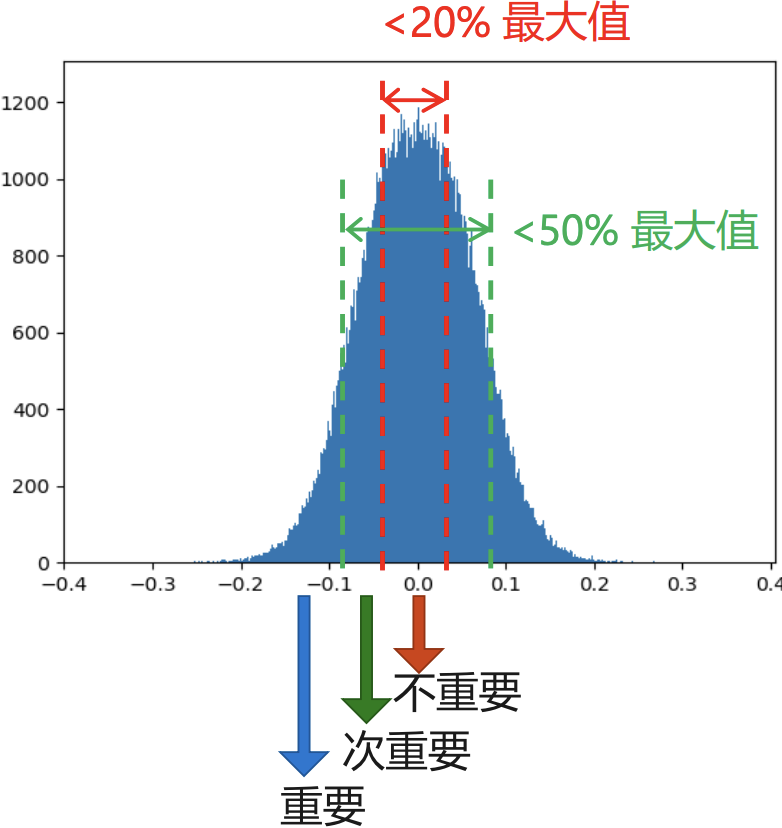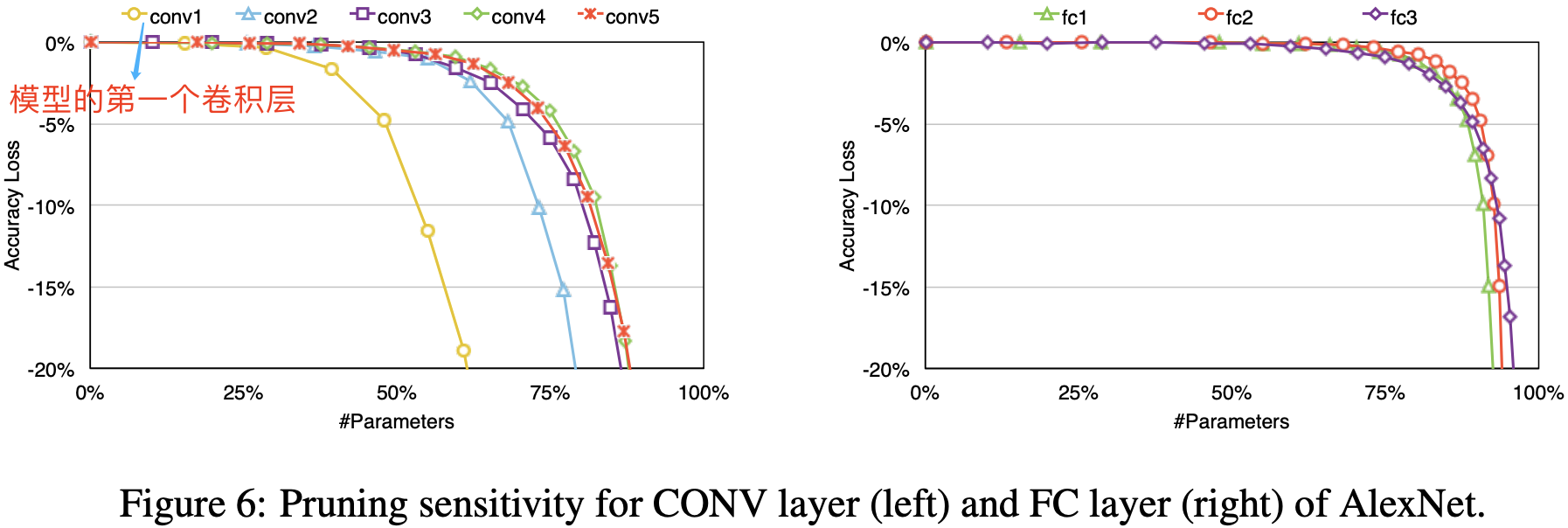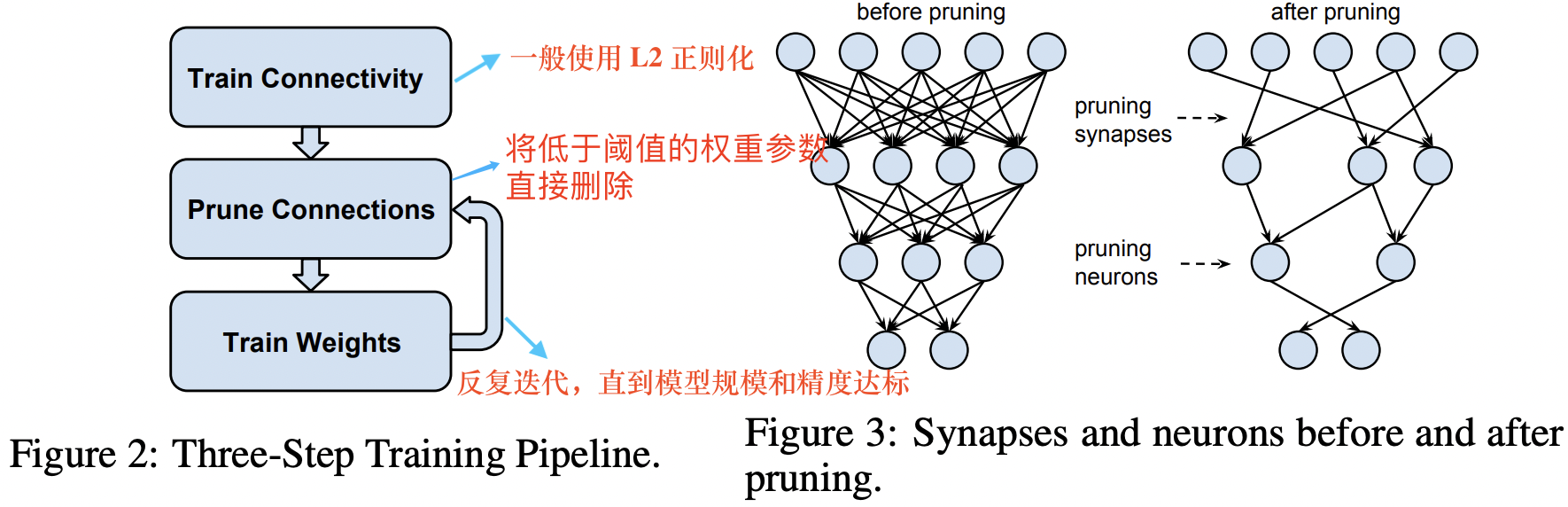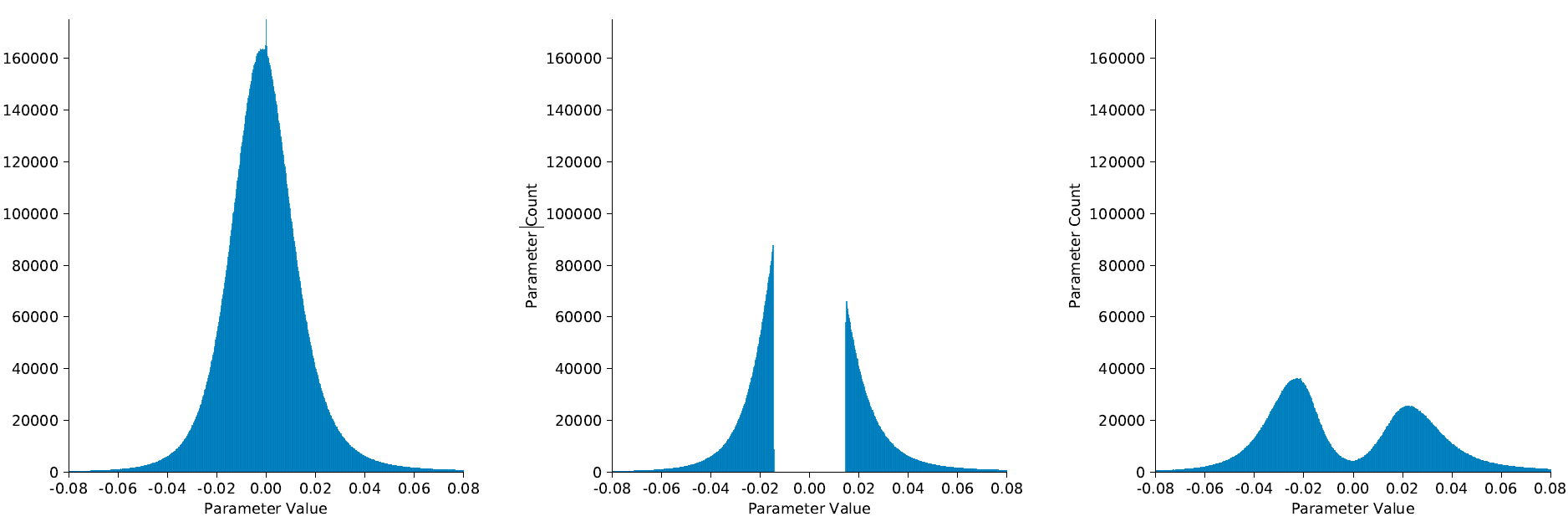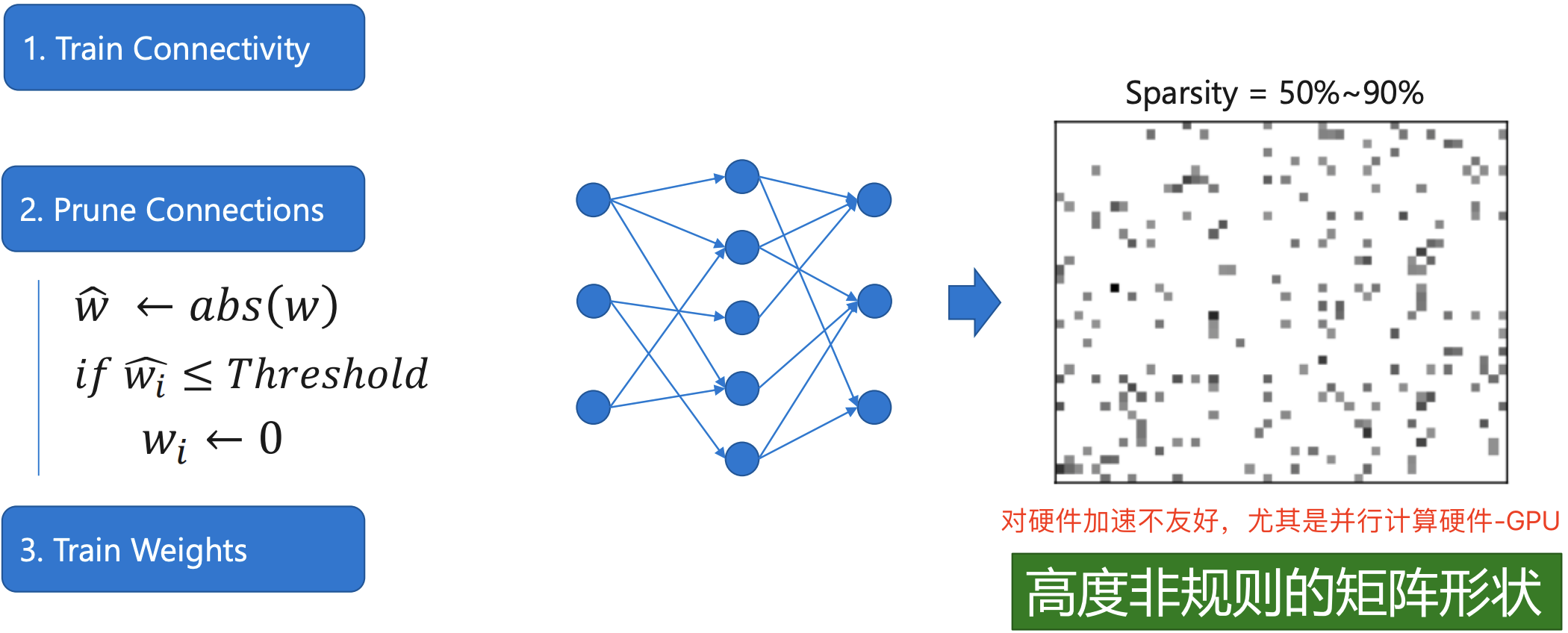### 2.2，激活稀疏

# cbr 组件示例代码
def convbn_relu(in_planes, out_planes, kernel_size, stride, pad, dilation):
return nn.Sequential(
nn.Conv2d(in_planes, out_planes,
kernel_size=kernel_size, stride=stride,
dilation=dilation, bias=False),
nn.BatchNorm2d(out_planes),
nn.ReLU(inplace=True)
)


ReLU 激活函数的定义为：

$$ReLU(x) = max(0, x)$$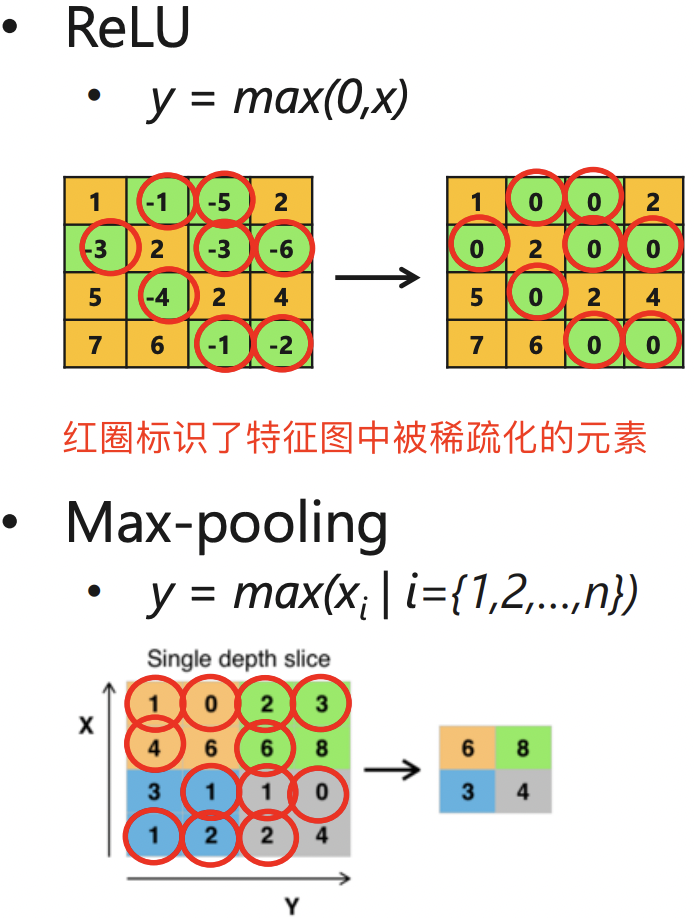$$APoZ^{(i)}c = APoZ(O_c^{(i)}) = \frac{\sum_k^N \sum_j^M f(O^{(i)}{c,j}(k=0))}{N \times M}$$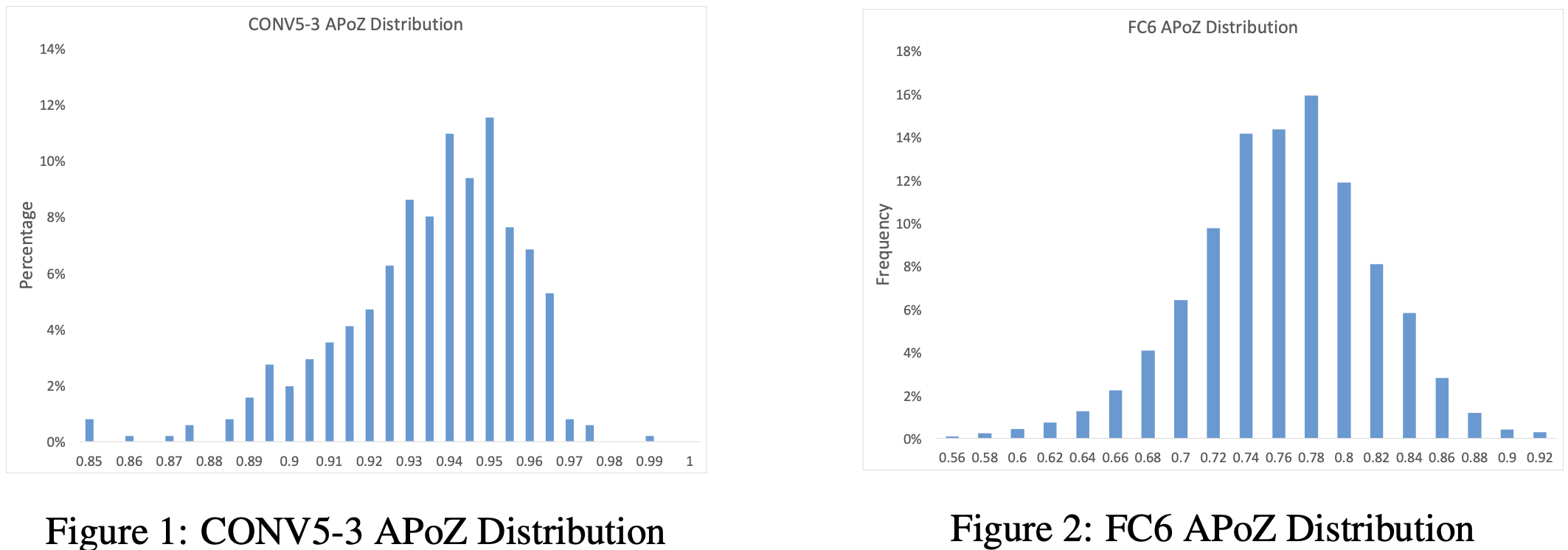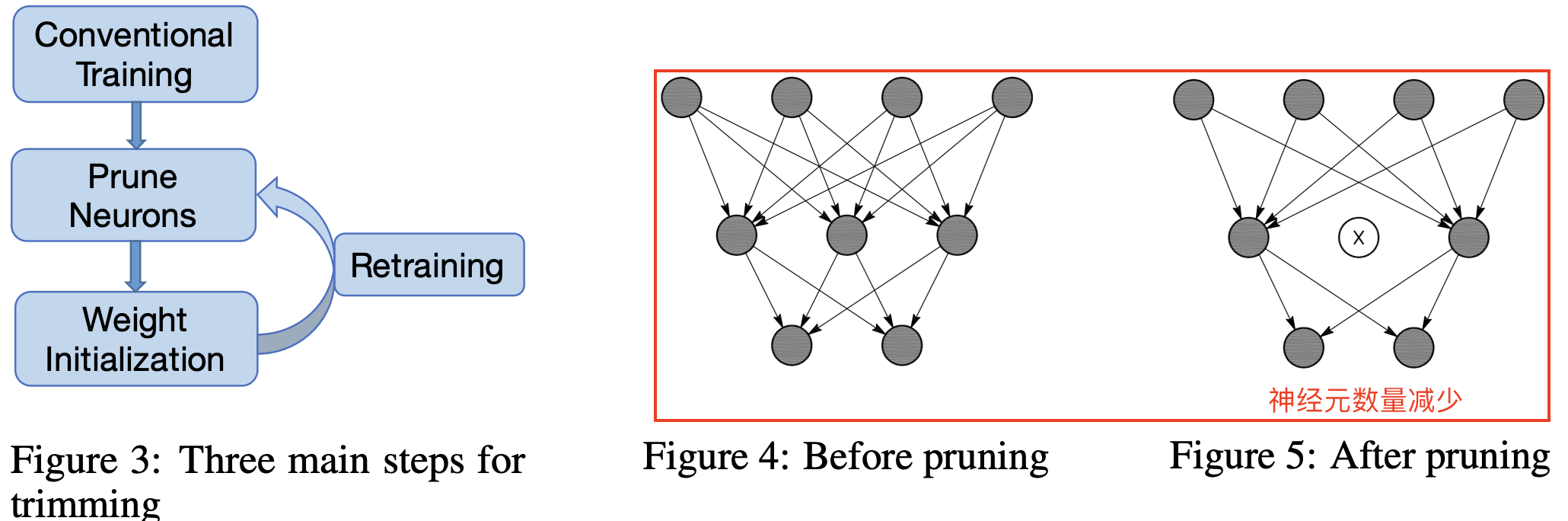1. 首先，网络在常规过程下进行训练，每层中的神经元数量根据经验设置。 接下来，我们在大型验证数据集上运行网络以获得每个神经元的 APoZ。
2. 根据特定标准修剪具有高 APoZ 的神经元。 当一个神经元被修剪时，与神经元的连接被相应地移除（参见上图右边红色框）。
3. 神经元修剪后，修剪后的网络使用修剪前的权重进行初始化。 修剪后的网络表现出一定程度的性能下降。因此，在最后一步中，我们重新训练网络以加强剩余的神经元以增强修剪后网络的性能。

### 2.3，梯度稀疏

AlexNet 模型的训练是采用分布式训练。深度神经网络训练中的各层梯度值存在高度稀疏特性。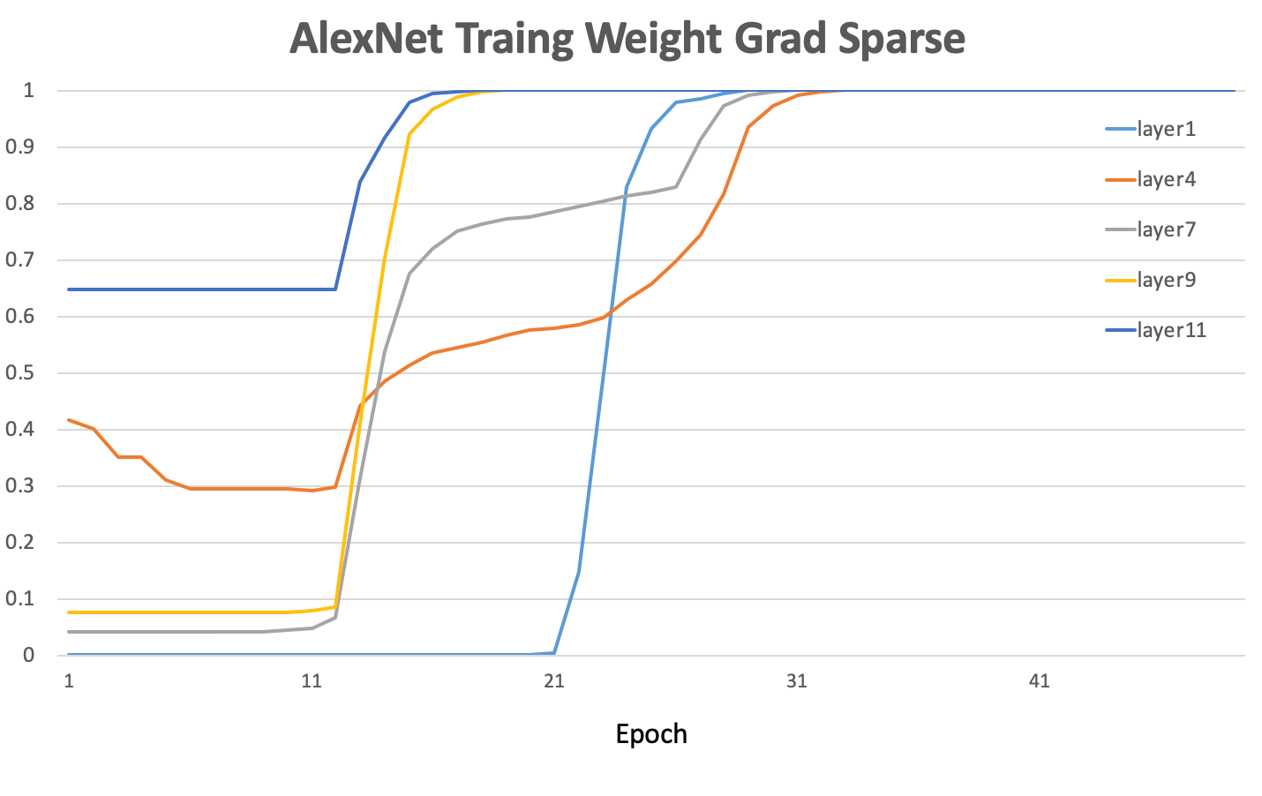1. 选择固定比例的正负梯度更新：在网络上传输根据一定比例选出的一部分正、负梯度更新值。Dryden 等人2016年的论文
2. 预设阈值：在网络上仅仅传输那些绝对值幅度超过预设阈值的梯度。Heafield (2017)论文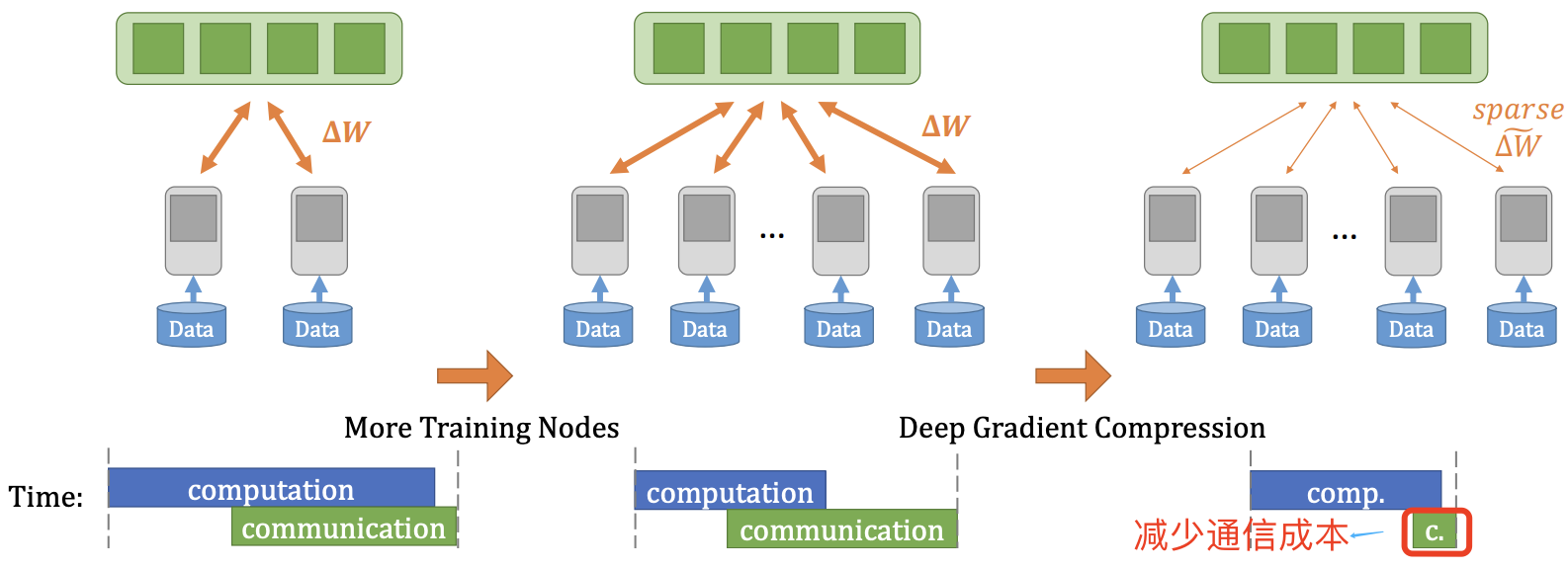### 2.4，小结

1. 早期的权重剪枝是非结构化的（细粒度稀疏）其对并行计算硬件-GPU支持并不友好，甚至可能完全没有效果，其加速效果需要在专用加速器硬件（一般是 ASIC）上实现，比如韩松提出的 EIE 加速硬件。
2. 更高层的网络冗余性更大，且卷积层的冗余性比全连接层的冗余性更少。所以 ResNet、MobileNet 等网络的剪枝难度大于 VGG、AlexNet 等。
3. 神经元剪枝相比权重剪枝更易损失模型精度，训练阶段的梯度则拥有最多的稀疏度。
4. 典型的模型剪枝三段式工作 pipeline 流程并不一定是准确的，最新的研究表明，对于随机初始化网络先进行剪枝操作再进行训练，有可能会比剪枝预训练网络获得更高的稀疏度和精度。此需要更多研究。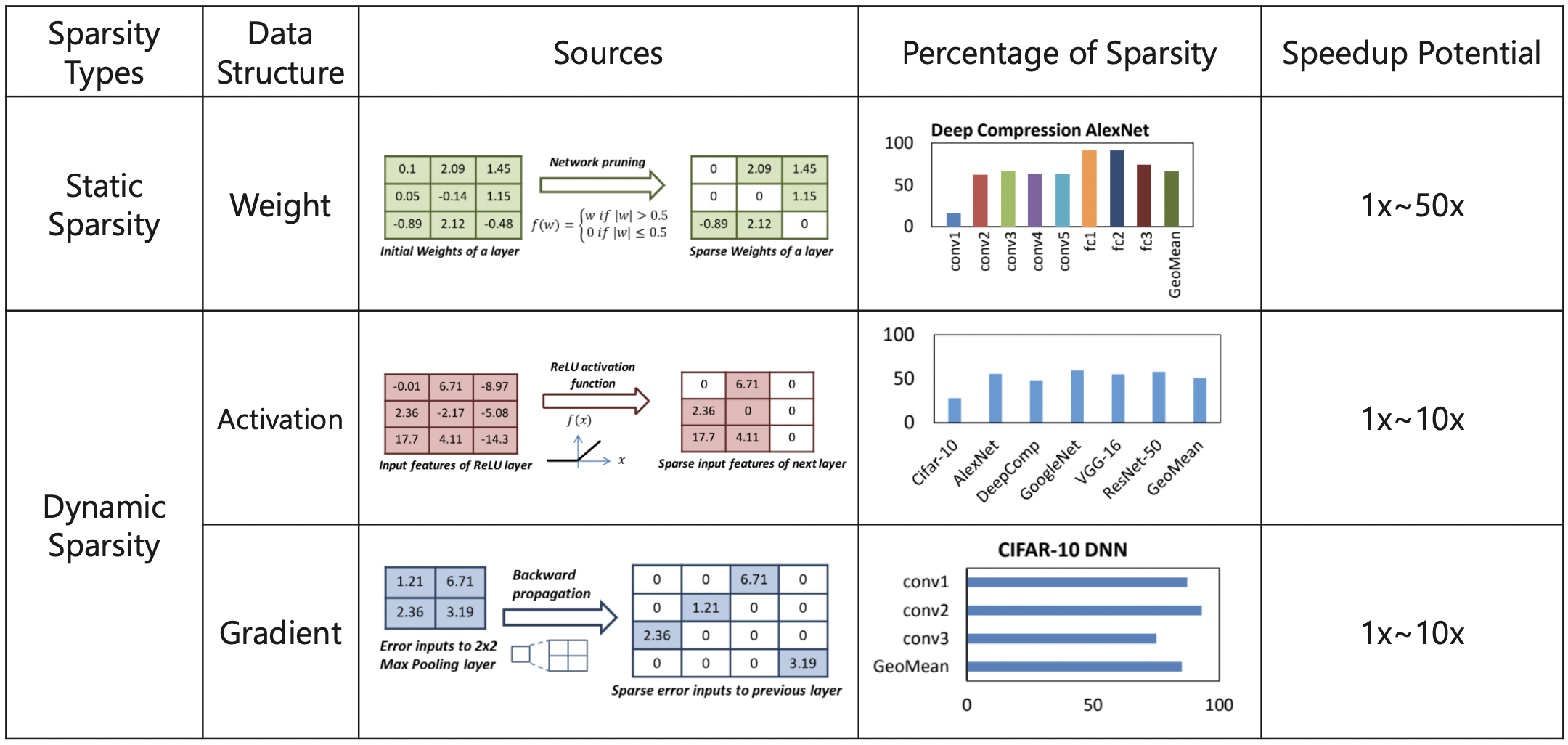## 三，结构化稀疏

### 3.1，结构化稀疏分类

#### 3.1.1，Channel/Filter 剪枝

channel 剪枝的工作是最多的，channel 剪枝和 filter 剪枝其实意义是一样的，一个过滤器移除了，对应输出 feature map 的一个通道自然也被移除，反之一样。

filter (channel) pruning (FP) 属于粗粒度剪枝（或者叫结构化剪枝 Structured Pruning），基于 FP 的方法修剪的是过滤器或者卷积层中的通道，而不是对个别权重，其原始的卷积结构不改变，所以剪枝后的模型不需要专门的算法和硬件就能够加速运行。

CNN 模型中通道剪枝的核心在于如何减少中间特征的数量，其中一个经典思路是基于重要性因子，即评估一个通道的有效性，再配合约束一些通道使得模型结构本身具有稀疏性，从而基于此进行剪枝。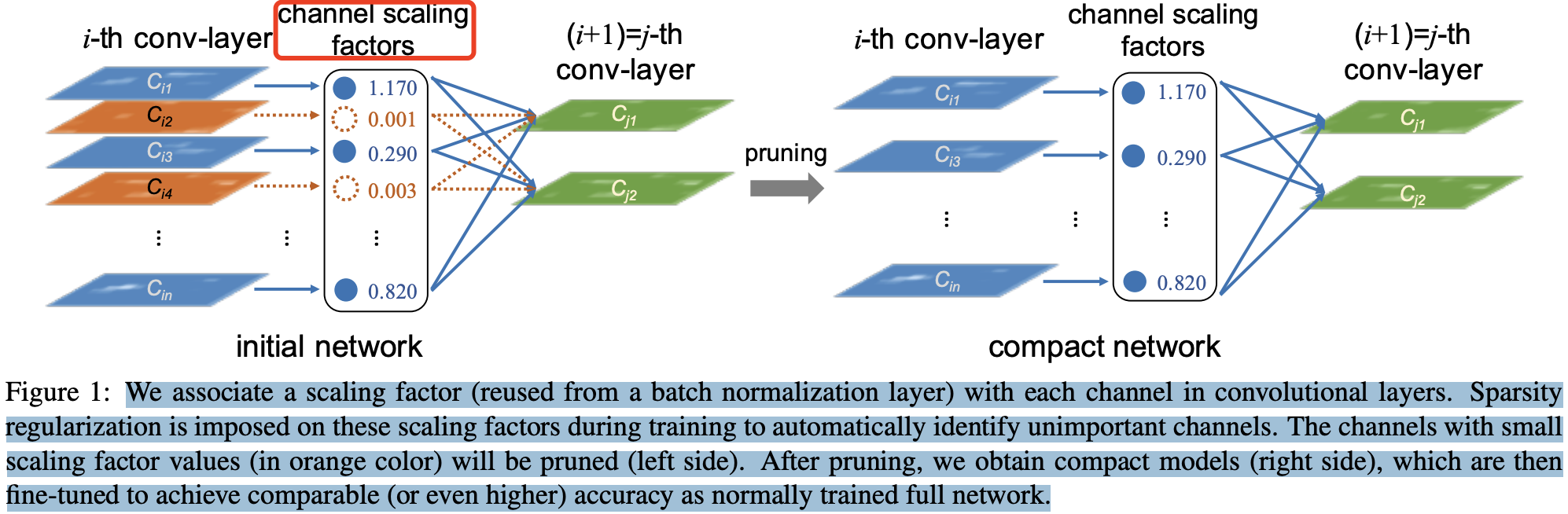BN 层的计算公式如下：

\begin{aligned} \mu_B &= \frac{1}{m}\sum_1^m x_i \ \sigma^2_B &= \frac{1}{m} \sum_1^m (x_i-\mu_B)^2 \ n_i &= \frac{x_i-\mu_B}{\sqrt{\sigma^2_B + \epsilon}} \ z_i &= \gamma n_i + \beta = \frac{\gamma}{\sqrt{\sigma^2_B + \epsilon}}x_i + (\beta - \frac{\gamma\mu_{B}}{\sqrt{\sigma^2_B + \epsilon}}) \end{aligned}

#### 3.1.2， 阶段级别剪枝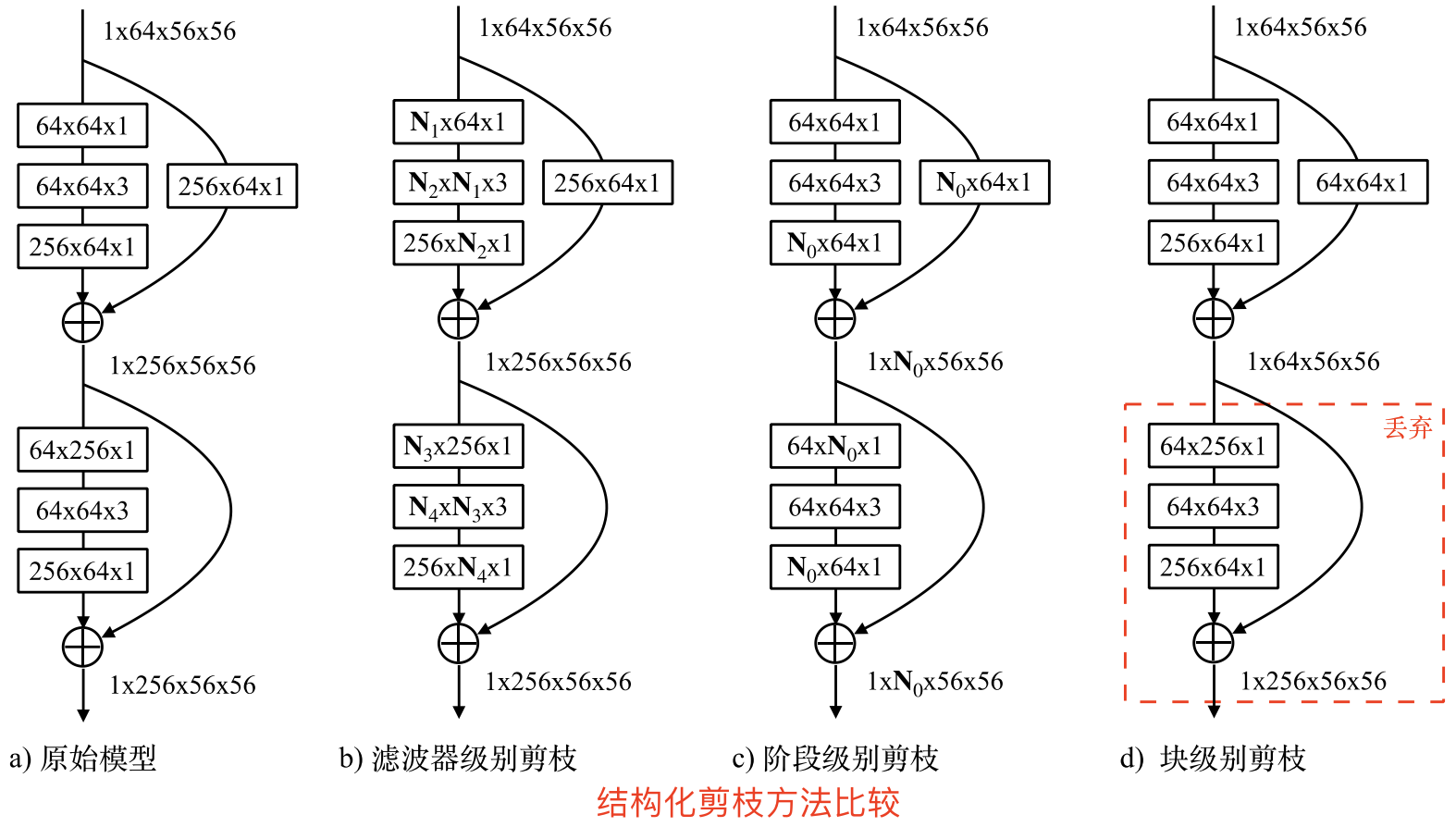### 3.2，结构化稀疏与非结构化稀疏比较

1. 非结构化稀疏具有更高的模型压缩率和准确性，在通用硬件上的加速效果不好。因为其计算特征上的“不规则”，导致需要特定硬件支持才能实现加速效果。
2. 结构化稀疏虽然牺牲了模型压缩率或准确率，但在通用硬件上的加速效果好，所以其被广泛应用。因为结构化稀疏使得权值矩阵更规则更加结构化，更利于硬件加速。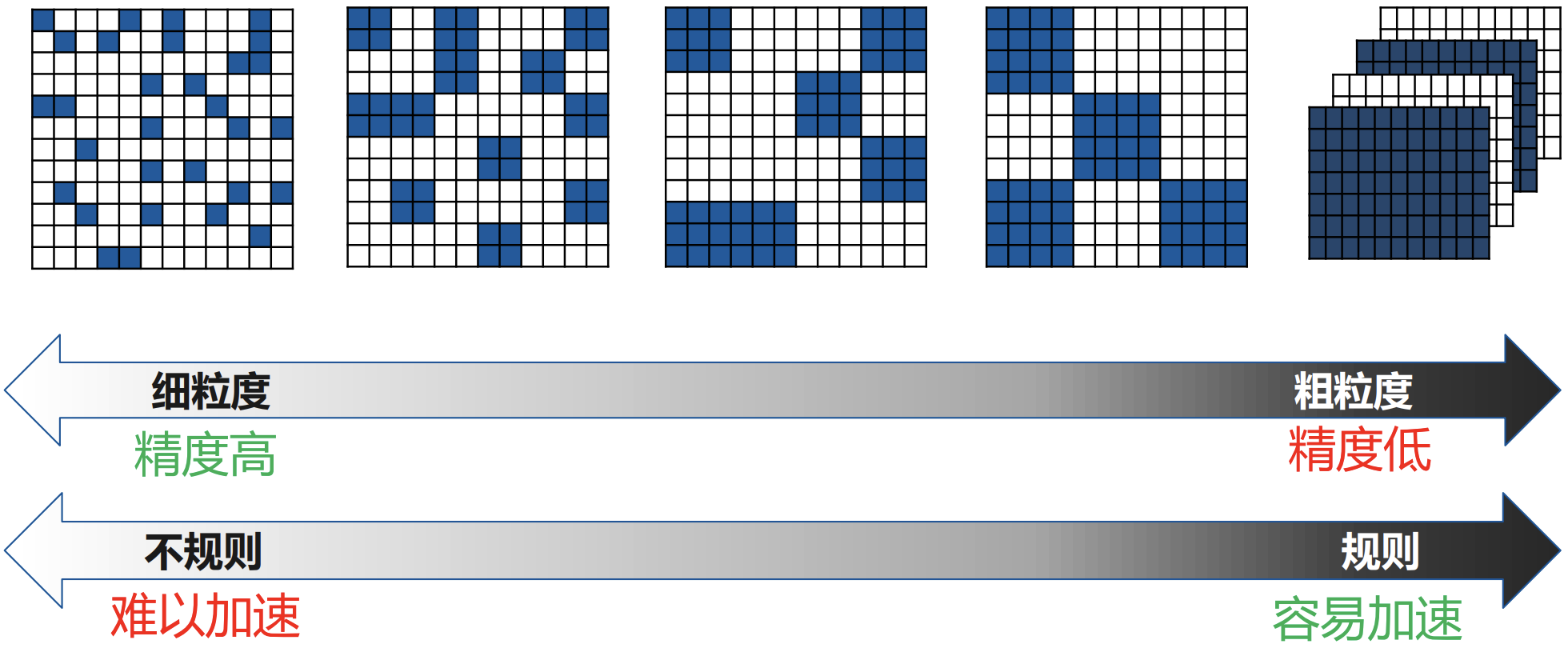## 参考资料